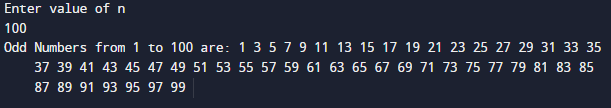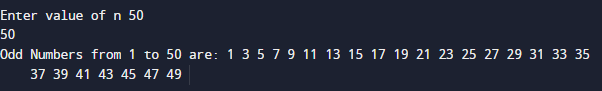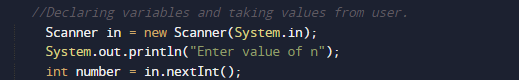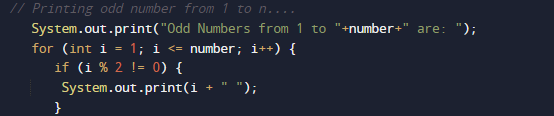# Java Program to display odd numbers from 1 to n or 1 to 100

In this tutorial you will learn about the Java Program to display odd numbers from 1 to n or 1 to 100 and its application with practical example.

In this tutorial, we will learn to create a Java Program to display odd numbers from 1 to n or 1 to 100 using Java programming.

## Prerequisites

Before starting with this tutorial we assume that you are best aware of the following Java programming topics:

• Java Operators.
• Basic Input and Output function in Java.
• Class and Object in Java.
• Basic Java programming.
• If-else statements in Java.
• For loop in Java.

## What is Odd Number.

A number is  called odd that number cannot be divided exactly into pairs or we can say odd numbers, when divided by 2, gives remainder 1. (5%2==1).

## Java Program to display odd numbers from 1 to n or 1 to 100

In this program we would print all odd number between “1 to n or 1 to 100” .first of we would take a value from user and find the Odd number between given numbers .let have a look at the code.

## Output

Odd number between 1-100.Odd number between 1-N. Value of n is 50.In the above program, we have first declared and initialized a set variables required in the program.

• number= it will hold entered number.
• i=   i for iteration.

After that we take a number from user and find odd number between the given number.Here we can use  for loops to display odd numbers:

• Using  for Loop
• Using if-else Statement.

In order to check  odd number, we divided the number from 1 to N by 2 if it leaves a remainder of 1,then the number is oddand the print the values.. As shown in image above.

In this tutorial we have learn about the Java Program to display odd numbers from 1 to n or 1 to 100 and its application with practical example. I hope you will like this tutorial.